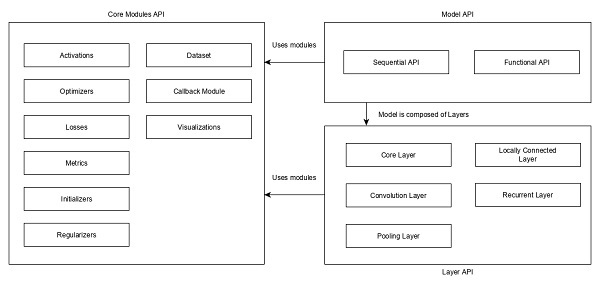# Keras - Deep learning

Keras provides a complete framework to create any type of neural networks. Keras is innovative as well as very easy to learn. It supports simple neural network to very large and complex neural network model. Let us understand the architecture of Keras framework and how Keras helps in deep learning in this chapter.

## Architecture of Keras

Keras API can be divided into three main categories −

• Model
• Layer
• Core Modules

In Keras, every ANN is represented by Keras Models. In turn, every Keras Model is composition of Keras Layers and represents ANN layers like input, hidden layer, output layers, convolution layer, pooling layer, etc., Keras model and layer access Keras modules for activation function, loss function, regularization function, etc., Using Keras model, Keras Layer, and Keras modules, any ANN algorithm (CNN, RNN, etc.,) can be represented in a simple and efficient manner.

The following diagram depicts the relationship between model, layer and core modules −Let us see the overview of Keras models, Keras layers and Keras modules.

## Model

Keras Models are of two types as mentioned below −

Sequential Model − Sequential model is basically a linear composition of Keras Layers. Sequential model is easy, minimal as well as has the ability to represent nearly all available neural networks.

A simple sequential model is as follows −

```from keras.models import Sequential
from keras.layers import Dense, Activation

model = Sequential()
model.add(Dense(512, activation = 'relu', input_shape = (784,)))
```

Where,

• Line 1 imports Sequential model from Keras models

• Line 2 imports Dense layer and Activation module

• Line 4 create a new sequential model using Sequential API

• Line 5 adds a dense layer (Dense API) with relu activation (using Activation module) function.

Sequential model exposes Model class to create customized models as well. We can use sub-classing concept to create our own complex model.

Functional API − Functional API is basically used to create complex models.

## Layer

Each Keras layer in the Keras model represent the corresponding layer (input layer, hidden layer and output layer) in the actual proposed neural network model. Keras provides a lot of pre-build layers so that any complex neural network can be easily created. Some of the important Keras layers are specified below,

• Core Layers
• Convolution Layers
• Pooling Layers
• Recurrent Layers

A simple python code to represent a neural network model using sequential model is as follows −

```from keras.models import Sequential
from keras.layers import Dense, Activation, Dropout model = Sequential()

model.add(Dense(512, activation = 'relu', input_shape = (784,)))
```

Where,

• Line 1 imports Sequential model from Keras models

• Line 2 imports Dense layer and Activation module

• Line 4 create a new sequential model using Sequential API

• Line 5 adds a dense layer (Dense API) with relu activation (using Activation module) function.

• Line 6 adds a dropout layer (Dropout API) to handle over-fitting.

• Line 7 adds another dense layer (Dense API) with relu activation (using Activation module) function.

• Line 8 adds another dropout layer (Dropout API) to handle over-fitting.

• Line 9 adds final dense layer (Dense API) with softmax activation (using Activation module) function.

Keras also provides options to create our own customized layers. Customized layer can be created by sub-classing the Keras.Layer class and it is similar to sub-classing Keras models.

## Core Modules

Keras also provides a lot of built-in neural network related functions to properly create the Keras model and Keras layers. Some of the function are as follows −

• Activations module − Activation function is an important concept in ANN and activation modules provides many activation function like softmax, relu, etc.,

• Loss module − Loss module provides loss functions like mean_squared_error, mean_absolute_error, poisson, etc.,

• Optimizer module − Optimizer module provides optimizer function like adam, sgd, etc.,

• Regularizers − Regularizer module provides functions like L1 regularizer, L2 regularizer, etc.,

Let us learn Keras modules in detail in the upcoming chapter.